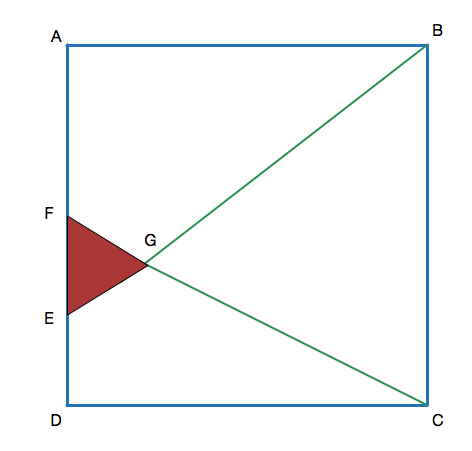# Problem 1 : Area in a square

Geometry Level 3Let $ABCD$ be a square with total area $1600$. $F$ is the midpoint of $AD$ and $E$ is the midpoint of $FD$. $BE$ and $CF$ intersect at $G$. Find the area of triangle $EFG$.

×